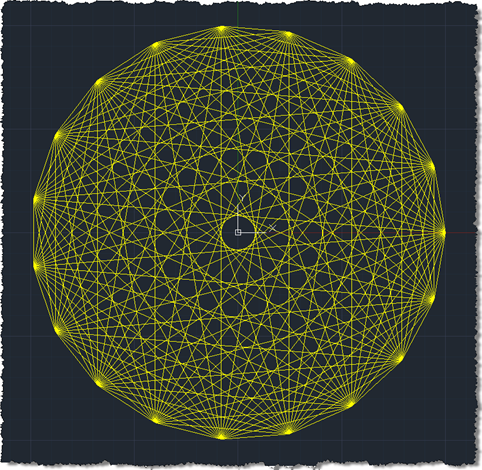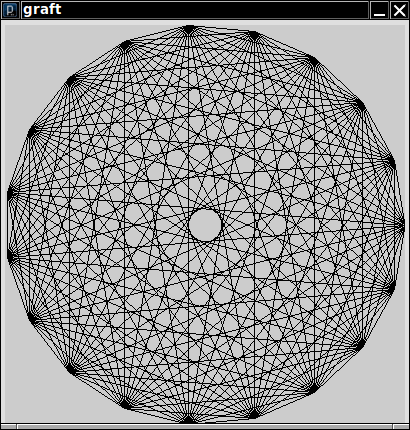# A Geometry Problem in Scala

I ran into this blog about making a pretty drawing in C# and F#.The task is to draw all lines between n evenly spaced points on a circle.

The solution in C# is surprisingly hard to read because it intermixes control flow with the details of the graphics processing. The author's attempt at a “functional” version just translated the iterations to recursion, and it wasn't really any simpler. I figured that I could do better in Scala. (Someone else contributed a much more functional version, and it is along the same lines as my Scala solution.)

Here is how I approached it.

First, I figured that I needed a way of getting all pairs (i,j) of numbers 0 ≤ i < j < n. That's easy.

`def pairs(n : Int) = for (i <- 0 until n; j <- 0 until n; if i < j) yield (i, j)`

For example, `pairs(4)` is

`Vector((0,1), (0,2), (0,3), (1,2), (1,3), (2,3))`

I also need to get the actual points.

The ith point of an n-gon on a unit circle is (cos(2πi / n), sin(2πi / n). If we aren't on a unit circle but in a square of a given width, then we need to transform, like this:

```def point(i : Int, n : Int, width : Int) =
(((cos(2 * Pi * i / n) + 1) * width / 2).toInt,
((sin(2 * Pi * i / n) + 1) * width / 2).toInt)```

All points are

`val points = for (i <- 0 until n) yield point(i, n, width)`

To get the lines, I have to look up the first and second point for each pair.

`val lines = for ((i, j) <- pairs(n)) yield (points(i), points(j))`

Finally, I need to draw them all. Let's assume that we can draw lines with a `line` function.

`for (((x1, y1), (x2, y2)) <- lines) line(x1, y1, x2, y2)`

In the nifty SPDE environment (a Processing clone for Scala), you can do just that. Here is the complete SPDE program:

```import math._

size(400, 400)

def pairs(n : Int) = for (i <- 0 until n; j <- 0 until n; if i < j) yield (i, j)

def point(i : Int, n : Int, width : Int) =
(((cos(i * 2 * Pi / n) + 1) * width / 2).toInt,
((sin(i * 2 * Pi / n) + 1) * width / 2).toInt)

def draw {
val n = 19
val points = for (i <- 0 until n) yield point(i, n, width)
val lines = for ((i, j) <- pairs(n)) yield (points(i), points(j))
for (((x1, y1), (x2, y2)) <- lines) line(x1, y1, x2, y2)
}```

Very nice: Two helper functions and three lines of data transformations. (Note how pattern matching in the `for` loop is our friend.)What does it all mean? Looking at the iterative solution, it seems as if the implementor was focused on getting the nested loops right. With Scala, I wanted to get the data right. I knew that, once I had the lines, I could call

`for (... <- lines) line(...) `

How could I get all the lines? I needed to get all the points. Then I needed to join them into pairs. Each of these steps is an easy transformation. So, the entire computation comes down to a sequence of data transformations.

Is this a reason to switch from Java or C# to Scala or F#? By itself, of course, it is not.

But now look at the Processing web site and all the nifty drawings that you can make with a few lines of Processing code. Nice, but you have to learn yet another domain-specific language. That's a waste. With Scala, you just learn one language. Together with SPDE, Scala becomes your DSL for drawing, and your investment is repaid.